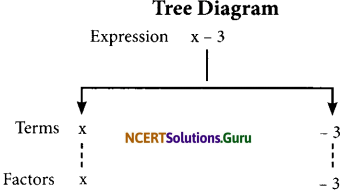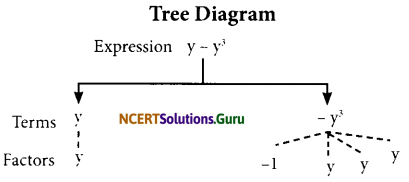# NCERT Solutions for Class 7 Maths Chapter 12 Algebraic Expressions Ex 12.1

These NCERT Solutions for Class 7 Maths Chapter 12 Algebraic Expressions Ex 12.1 Questions and Answers are prepared by our highly skilled subject experts.

## NCERT Solutions for Class 7 Maths Chapter 12 Algebraic Expressions Exercise 12.1

Question 1.
Get the algebraic expressions in the following cases using variables, constants and arithmetic operations.
(i) Subtraction of z from y.
(ii) One-half of the sum of numbers x and y.
(iii) The number z multiplied by itself.
(iv) One-fourth of the product of numbers p and q.
(v) Numbers x and y both squared and added.
(vi) Number 5 added to three times the product of number m and n.
(vii) Product of numbers y and z subtracted from 10.
(viii) Sum of numbers a and b subtracted from their product.
(i) y – z
(ii) $$\frac { 1 }{ 2 }$$(x + y)
(iii) z2
(iv) $$\frac { 1 }{ 4 }$$pq
(v) x2 + y2
(vi) 5 + 3mn
(vii) 10 – yz
(viii) ab – (a + b)Question 2.
(i) Identify the terms and their factors in the following expressions. Show the terms and factors by tree diagrams.
(a) x – 3
(b) 1 + x + x2
(c) y – y3
(d) 5xy2 + 7x2y
(e) – ab + 2b2 – 3a2

(ii) Identify terms and factors in the expressions given below:
(a) – 4x + 5
(b) – 4x + 5y
(c) 5y + 3y2
(d) xy + 2x2y2
(e) pq + q
(f) 1.2ab- 2.4 b + 3.6a
(g) $$\frac { 3 }{ 4 }$$x + $$\frac { 1 }{ 4 }$$
(h) 0.1p2 + 0.2p2
(i) (a) x – 3
Terms : x; – 3 Factors x; -3
1 + x + x2(b) 1 + x + x2
Terms = 1; x; x2
Factors = 1; x; x, x (x2 )(c) y-y3
Terms: y; -y3
Factors y;-1,y,y,y(d) 5xy2 + 7x2 y
Terms: 5xy2; 7x2 y
Factors: 5, x, y, y; 7, x, x, y(e) -ab + 2b2 – 3a2
Terms: -ab; 2b2, -3a2
Factors: -1, a, b; 2; b; b; 3, a, a(ii)

 Expression Terms Factors (a) -4x + 5 -4x 5 -4x and z 5 and y (b) – 4x + 5y -4x 5y – 4 and z 5 and y (c) 5y + 3y2 5y 3y2 5 and y 3, y and y (d) xy + 2x2 y2 Xy 2x2y2 x and y 2, x.x, y and y (e) pq + q Pq q p and q q (f) 1.2ab – 2.4 b + 3.6a 1.2ab – 2.4 b 3.6a 1.2, a and b. -2.4 and b 3.6 and a (g) $$\frac{3}{4}$$x + $$\frac{1}{4}$$ $$\frac{3}{4}$$x $$\frac{1}{4}$$ $$\frac{3}{4}$$ and x $$\frac{1}{4}$$ (h) 0.lp2 + 0.2q2 0.1p2 0.2q2 0.1, p,p 0.2, q and q

Question 3.
Identify the numerical coefficients of terms (other than constants) in the following expressions:
(i) 5 – 3t2
(ii) 1 + t + t2 + t3
(iii) x + 2xy + 3y
(iv) 100m + 1000n
(v) -p2q2 + 7pq
(vi) 1.2a + 0.8b
(vii) 3.14r2
(viii) 2(l + b)
(ix) 0.1y + 0.01y2

 Expression Terms (other than constant) Numerical coefficient (i) 5 – 3t2 – 3t2 -3 (ii) 1 + t + t2 + t3 t t2 t3 1 1 1 (iii) x + 2xy + 3y x 2xy 3y 1 2 3 (iv) 100 m + lOOOn 100m 1000n 100 1000 (v) -p2q2 + 7pq -p2q2 7pq -1 7 (vi) 1.2a + 0.8b 1.2a 0.8b 1.2 0.8 (vii) 3.14r2 3.14r2 3.14 (viii) 2(l + b) 2l 2b 2 2 (ix) 0.1y + 0.01y2 0.1y 0.01y2 0.1 0.01Question 4.
(a) Identify terms which contain x and give the coefficient of x.
(i) y2x + y
(ii) 13y2 – 8yx
(iii) x + y + 2
(iv) 5 + z + zx
(iv) 1+x + xy
(vi) 12xy2 + 25
(vii) 7x + xy2

(b) Identify terms which contain y2 and give the coefficient of y2.
(i) 8-xy2
(ii) 5y2 + 7x
(iii) 2x2y – 15xy2 + 7y2

 Expression Term containing x Coefficient of x (i) y2x + y y2x y2 (ii) x + y + 2 – 8yx – 8y (iii) 5 + z + zx x l (iv) 5 + z + zx zx Z (v) 1 + x + xy x xy 1 y (vi) 12xy2+ 25 12xy2 12y2 (vii) 7x + xy2 7x xy2 7 y2

(b)

 Expression Term containing y2 Coefficient of y2 (i) 8 – xy2 -xy2 -X (ii) 5y2 + 7x 5y2 5 (iii) 2x2y – 15xy2 + 7y2 – 15xy2 7f – 15x 7

Question 5.
Classify into monomials, binomials and trinomials.
(i) 4y – 7z
(ii) y2
(iii) x + y – xy
(iv) 100
(v) ab – a – b
(vi) 5 – 3t
(vii) 4p2q – 4pq2
(viii) 7mn
(ix) z2 – 3z + 8
(x) a2 + b2
(xi) z2 + z
(xii) 1 + x + x2
(i) 4y – 7z
The expression 4y – 7z is having two unlike terms (4y and – 7z)
∴ It is a binomial.(ii) y2
The expression y2 is having only one term (y2)
∴ It is a monomial.

(iii) x + y – xy
The expression x + y – xy is having three terms (x, y and – xy)
∴ It is a trinomial.

(iv) 100
The expression 100 is having only one term (100)
∴ It is a monomial.

(v) ab – a – b.
The expression ab – a – b is having three terms (ab, -a, and -b)
∴ The expression is a trinomial.

(vi) 5 – 3t
The expression 5 – 3t is having two terms (5 and -3t)
∴ It is a binomial expression.

(vii) 4p2q – 4pq2
The expression 4p2q – 4pq2 is having
two unlike terms (4p2q and – 4pq2)
∴ The expression is a binomial.

(viii) 7mn
The expression 7mn is having only one term (ie 7mn)
∴ The expression is a monomial.

(ix) z2– 3z + 8
The expression z2 – 3z + 8 is having three terms (ie z2, – 3z and 8)
∴ The expression is a trinomial.(x) a2 + b2
The expression (a2 + b2) is having two unlike terms (a2 and b2)
∴ It is a binomial expression.

(xi) z2 + z
The expression z2 + z is having two unlike terms (z2 and z)
∴ The expression in binomial.

(xii) 1 + x + x2
The expression 1 + x + x2 is having three terms (1, x and x2)
∴ The expression is a trinomial.

Question 6.
State whether a given pair of terms is of like or unlike terms.
(i) 1, 100
(ii) -7x, $$\frac { 5 }{ 2 }$$x
(iii) -29x, -29y
(iv) 14xy, 42yx
(v) 4m2p, 4mp2
(vi) 12xz, 12x2z2
(i) 1, 100 is a pair of like terms.
(ii) $$\frac { 5 }{ 2 }$$x is a pair of like terms.
(iii) – 29x, – 29y is a pair of unlike terms.
(iv) 14xy, 42yx is a pair of like terms.
(v) 4m2p, 4mp2 is a pair of unlike terms.
(vi) 12xz; 12x2z2 is a pair of unlike terms.Question 7.
Identify like terms in the following:
(a) -xy2, – 4yx2, 8x2, 2xy2, 7y, -11x2, 100x, -11yx, 20x2y, – 6x2, y, 2xy, 3x
(b) 10pq, 7p, 8q, – p2q2, -7pq, -100q, -23, 12q2p2, -5p2, 41, 2405p, 78qp,
13p2q, qp2,701p2